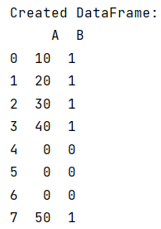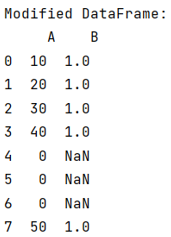# How to modify a subset of rows in a pandas DataFrame?

Given a Pandas DataFrame, we have to modify a subset of rows.
Submitted by Pranit Sharma, on June 13, 2022

Sometimes, we need to modify a column value based upon another column value. For example, if you have two columns 'A' and 'B', and you want the value of 'B' to be Nan whenever the value of 'A' becomes 0. This can be done with the help of the pandas.DataFrame.loc property .

To work with pandas, we need to import pandas package first, below is the syntax:

```import pandas as pd
```

Let us understand with the help of an example,

```# Importing pandas package
import pandas as pd

# Importing numpy package
import numpy as np

# Creating a dictionary
d = {
'A':[10,20,30,40,0,0,0,50],
'B':[1,1,1,1,0,0,0,1]
}

# Creating a DataFrame
df = pd.DataFrame(d)

# Display DataFrame
print("Created DataFrame:\n",df,"\n")
```

Output:Now, we will use the loc property for modifying a column value, suppose we want a value to be set for a column whenever a certain condition is met for another column, we can use the following concept:

```df.loc[selection criteria, columns I want] = value
```

Let us observe the following code snippet,

## Python code to modify a subset of rows

```# Applying condition and modifying
# the column value
df.loc[df.A==0, 'B'] = np.nan

# Display modified DataFrame
print("Modified DataFrame:\n",df)
```

Output:Preparation

What's New

Top Interview Coding Problems/Challenges!